Open in App
Not now

# Equations of Lines Parallel to the x-axis and y-axis

• Last Updated : 24 Nov, 2021

Linear Equations allow us to explain a lot of physical phenomena happening around us. For example, A train running between two stations at a constant speed, the speed of a falling object. Even the straight lines we draw on paper can be represented in form of linear equations mathematically. A linear equation in two variables can be represented on a 2D graph as a straight line. Let’s look at them in a formal way.

### Linear Equation in Two Variables

Linear equation in two variables are represented as,

ax + by + c = 0

Here a, b, and c are real numbers. a and b both should not be zero.

Solutions to the linear equations

Solutions to the linear equations are those points (x, y) that satisfy the given equation. There are infinitely many possible solutions to a linear equation. While solving linear equations we must keep a few things in mind. The solution of a linear equation is not affected when,

1. The same number or term is added or subtracted from both sides of the equation.
2. We multiply the same numbers to both sides of the equation.

Question: Find out different solutions to the equation y + 2x = 4.

Solution:

To find out the different solutions in general. Let’s assume one variable and find out the value of other variables.

Let’s say x = 0, then

y + 2.0 = 4

⇒ y = 4.

Again let’s say x = 2, then

y + 2.2 = 4

⇒ y = 0

Similarly, for x = 3, y = -2.

So, like this many solutions can be found for the given linear equation.

Let’s see now how to plot these solutions on the graph.

### Plotting the Solutions on the Graph

The solutions to the linear equations in two variables make a straight line on the graph. There are some types of patterns observed in the plots of linear equations. These linear equations sometimes pass through the origin, sometimes are parallel to the x-axis or y-axis and most of the time are skewed. We will look at all these cases with examples

Let’s say we have an equation y = 4x – 5. Let’s plot it

Putting x = 0, we get y = -5. Similarly, let’s put different values of x and figure out the values of y. After finding out sufficient values, we will join all of them to make a straight line.

The graph for this equation looks like,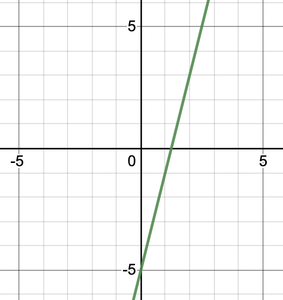### Equation of Lines parallel to x-axis and y-axis

Sometimes linear equations when plotted on a graph become parallel to either the x-axis or y-axis. When does that happen? And what does it mean?

For a general equation of the form

y – k = 0

or

x – k = 0

Where k is any real constant.

In one dimensional plane, these equations will represent a point x = k or y = k. But when they are brought and represented as a linear equation in two variables, they represent lines parallel to axes. Let’s look at how does this happen.

x – 2 when represented as an equation in two variables will look like x + 0.y – 2 = 0. Let’s find the solutions to this equation and plot them. Notice that the equation won’t be affected by any value of y. So, all the values of y can be included in the equation. The only value of x that satisfies the equation is x = 2.

Thus,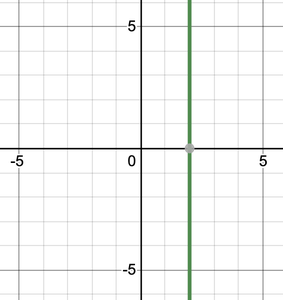Similar analysis can be done for the equation y – 2 = 0 where values of x don’t affect the equation thus all the values of x can be included in the equation.

Thus,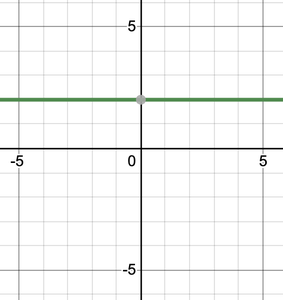### Equations Passing through Origin

Some equations have (0, 0) as a solution. The plot for such equations will always pass through the origin. For example:

y = 2x

(0,0) satisfies these equations, which means it is one of the solutions and the graph of this equation now must pass through the origin. Let’s plot its graph by finding out other solutions.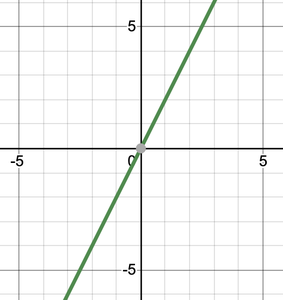Notice that these equations are in the form

y = kx

The constant ‘c’ is always zero in this case. If it’s not zero, these equations cannot have (0, 0) as a solution.

Let’s look at sample problems related to these concepts we studied till now.

### Sample Problems

Question 1: Give geometric representation of 5x + 2y = 10.

Let’s find out the solutions to this equation. Assume values for one variable, reduce the equation to a single variable form and then find out the value for other variable.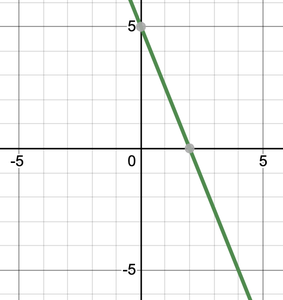Question 2: Give geometric representation for x = 10.

Solution:

As explained in the previous sections, this can be represented as,

x + 0.y = 10

Now for x = 10 and any other value of “y” it will work. So, the solutions are (10,y) where y can be anything.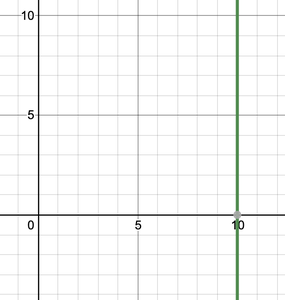Question 3: Plot the graph for the equation 4x = 2y.

Solution:

Let’s find out the solutions to this equation. Assume values for one variable, reduce the equation to a single variable form and then find out the value for other variable.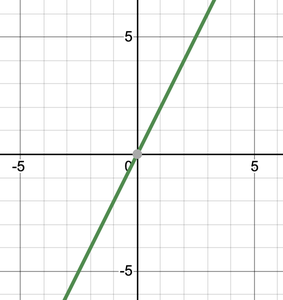Question 4: We have an equation 3x + ay = 12. It is known that (2, 3) is a solution to this equation. Find out the value of a.

Solution:

The solution the equation should satisfy it. (2,3) is a solution.

3(2) + a(3) = 12

⇒ 6 + 3a = 12

⇒ 3a = 6

⇒ a = 2.

Question 5: We know that the force applied to the body is directly proportional to its acceleration. The proportional is given by the mass of the body. Let’s say have a body of 5Kg. Plot the Force and acceleration curve for this body.

Solution:

It’s given that force applied to the body is directly proportional to its acceleration. Let’s say “F” is the force applied and “a” is the acceleration.

F = ka

Here,”k” is the constant of proportionality which is given by the mass of the body.

So, the equation becomes

F = 5a

Now let’s plot the solutions to this equation,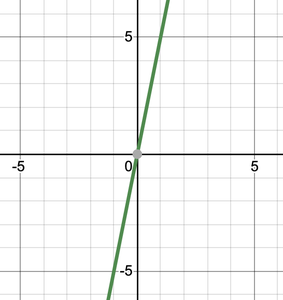Question 6: Give the geometric representation of y = 5 as

1. One variable equation
2. Two variable equation

Solution:

1. One Variable equation.

y = 5 has only one solution, y = 5. It can be represented on number line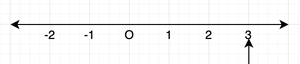2. Two Variable Equation

y = 5 can be represented as 0.x + y = 5.

Plotting the solutions to this,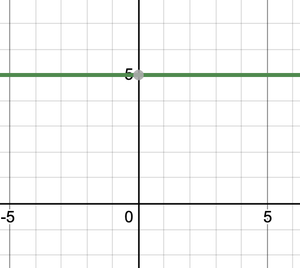My Personal Notes arrow_drop_up
Related Articles